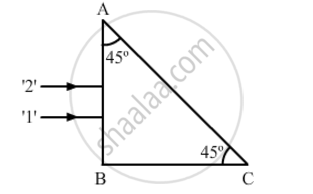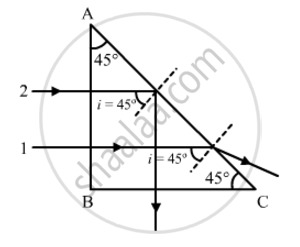# Two Monochromatic Rays of Light Are Incident Normally on the Face Ab of an Isosceles Right-angled Prism Abc. the Refractive Indices of the Glass Prism for the Two Rays '1' and '2' Are Respectively 1.3 and 1.5. Trace the Path of These Rays After Entering the Prism. - Physics

Two monochromatic rays of light are incident normally on the face AB of an isosceles right-angled prism ABC. The refractive indices of the glass prism for the two rays '1' and '2' are respectively 1.3 and 1.5. Trace the path of these rays after entering the prism.#### Solution

Critical angle of ray 1:

sin(c_1)=1/mu_1=1/1.3

=>c_1=sin^(-1)(1/1.3)=50.35^@

Similarly, critical angle of ray 2:

sin(c_2)=1/mu_0=1/1.52

=>c_2=sin^(-1)(1/1.52)=41.14^@

Both the rays will fall on the side AC with angle of incidence (i) equal to 45°. Critical angle of ray 1 is greater than that of i. Hence, it will emerge from the prism as shown in the figure. Critical angle of ray 2 is less than that of i. Hence, it will be internally reflected as shown in the figureConcept: Refraction of Monochromatic Light
Is there an error in this question or solution?
2013-2014 (March) All India Set 2

Share